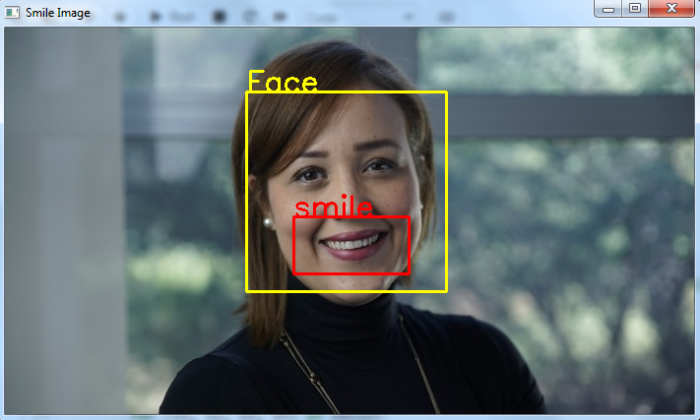# Smile detection using haar cascade in OpenCV using Python

We will use the Haar cascade classifier for smile detection in an image. A haar cascade classifier is an effective object detection method. It is a machine learning based approach. To train a haar cascade classifier for smile detection, the algorithm initially needs a lot of positive images (images with smile) and negative images (images without smile). Then the classifier is trained from these positive and negative images. It is then used to detect smiles in other images.

We can use already trained haar cascades for smile detection. For smile detection in the input image, we need two haar cascades one for face detection and other for smile detection. We will use the following two haar cascades −

## Steps

To detect smile in an image, you can follow the steps given below −

• Import the required library. In all the following examples, the required Python library is OpenCV. Make sure you have already installed it.

• Read the input image using cv2.imread(). Specify the full image path. Convert the image to grayscale image.

• Detect faces in the input image using face_cascade.detectMultiScale(). It returns the coordinates of detected faces in (x,y,w,h) format.

• Define face roi as image[y:y+h, x:x+w] for the detected face. Now detect the smile within the detected face area (roi). Use smile_cascade.detectMultiScale(). It also returns the coordinate of the bounding rectangle of eyes in (sx,sy,sw,sh) format.

• Draw the bounding rectangles around the detected smile (mouth) in the original image using cv2.rectangle().

• Display the image with the drawn bounding rectangles around the mouth.

Let's have a look at some examples for more clear understanding.

## Example

In this Python program, we perform smile detection in the input image using haar cascade.

# import required libraries
import cv2

# convert the image to grayscale
gray = cv2.cvtColor(img, cv2.COLOR_BGR2GRAY)

# Detects faces in the input image
print('Number of detected faces:', len(faces))

# loop over all the faces detected
for (x,y,w,h) in faces:

# draw a rectangle in a face
cv2.rectangle(img,(x,y),(x+w,y+h),(0,255,255),2)
cv2.putText(img, "Face", (x, y), cv2.FONT_HERSHEY_SIMPLEX, 1, (0, 255, 255), 2)
roi_gray = gray[y:y+h, x:x+w]
roi_color = img[y:y+h, x:x+w]

# detecting smile within the face roi
if len(smiles) > 0:
print("smile detected")
for (sx, sy, sw, sh) in smiles:
cv2.rectangle(roi_color, (sx, sy), ((sx + sw), (sy + sh)), (0, 0, 255), 2)
cv2.putText(roi_color, "smile", (sx, sy),
cv2.FONT_HERSHEY_SIMPLEX, 1, (0, 0, 255), 2)
else:
print("smile not detected")

# Display an image in a window
cv2.imshow('Smile Image',img)
cv2.waitKey(0)
cv2.destroyAllWindows()


We will use this image as the Input File for this program −## Output

On execution, it will produce the following output −

Number of detected faces: 1
smile detected


And we get the following output window −